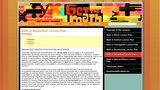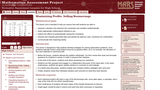Updating search results...

# 12 Results

View
Selected filters:
• WI.Math.Content.HSA-REI.A.1Conditional Remix & Share Permitted
CC BY-NC-SA
Rating
0.0 stars

This is a task from the Illustrative Mathematics website that is one part of a complete illustration of the standard to which it is aligned. Each task has at least one solution and some commentary that addresses important asects of the task and its potential use. Here are the first few lines of the commentary for this task: The product of two positive numbers is 9. The reciprocal of one of these numbers is 4 times the reciprocal of the other number. What is the sum of the ...

Subject:
Mathematics
Material Type:
Activity/Lab
Provider:
Illustrative Mathematics
Provider Set:
Illustrative Mathematics
Author:
Illustrative Mathematics
10/14/2013Conditional Remix & Share Permitted
CC BY-NC-SA
Rating
0.0 stars

This is a task from the Illustrative Mathematics website that is one part of a complete illustration of the standard to which it is aligned. Each task has at least one solution and some commentary that addresses important asects of the task and its potential use. Here are the first few lines of the commentary for this task: The following is a student solution to the inequality \frac{5}{18} - \frac{x-2}{9} \leq \frac{x-4}{6}. \begin{align} \frac{5}{18} - \frac{x-2}{9} & \le...

Subject:
Mathematics
Material Type:
Activity/Lab
Provider:
Illustrative Mathematics
Provider Set:
Illustrative Mathematics
Author:
Illustrative Mathematics
09/11/2013Restricted Use
Rating
0.0 stars

This website provides a visual resource for solving linear equations using a balance.

Subject:
Mathematics
Material Type:
Activity/Lab
Interactive
Simulation
Provider:
Utah State University
06/16/2015Conditional Remix & Share Permitted
CC BY-NC-SA
Rating
0.0 stars

Students connect polynomial arithmetic to computations with whole numbers and integers.  Students learn that the arithmetic of rational expressions is governed by the same rules as the arithmetic of rational numbers.  This unit helps students see connections between solutions to polynomial equations, zeros of polynomials, and graphs of polynomial functions.  Polynomial equations are solved over the set of complex numbers, leading to a beginning understanding of the fundamental theorem of algebra.  Application and modeling problems connect multiple representations and include both real world and purely mathematical situations.

Subject:
Algebra
Material Type:
Module
Provider:
New York State Education Department
Provider Set:
EngageNY
05/14/2013Restricted Use
Rating
0.0 stars

Digital algebra tiles that can be used on grids, dot grids, expression mats, equation mats, comparison mats, cornerpieces. Has x, y, x, y, xy, and unit tiles. This is not a lesson to use, but only the resource that can be used.

Subject:
Mathematics
Material Type:
Activity/Lab
Provider:
College Preparatory Mathematics
03/08/2017
RemixConditional Remix & Share Permitted
CC BY-NC-SA
Rating
0.0 stars

This will help students understand why it is important to check your work after you complete a math problem. They will be searching other students work to find a mistake in the work they completed.

Subject:
Education
Mathematics
Material Type:
Lesson
Author:
Lynn Aprill
Nicholas Schaumberg
09/09/2019
RemixConditional Remix & Share Permitted
CC BY-NC-SA
Rating
0.0 stars

This lesson will help students understand why it is important to check their work after they complete a math problem. They will be searching other students' work to find a mistake in the work they completed.

Subject:
Education
Mathematics
Material Type:
Lesson
Author:
Lynn Aprill
Nicholas Schaumberg
08/30/2019
RemixConditional Remix & Share Permitted
CC BY-NC-SA
Rating
0.0 stars

This will help students understand why it is important to check your work after you complete a math problem. They will be searching other students work to find a mistake in the work they completed.

Subject:
Education
Material Type:
Lesson
Author:
Nicholas Schaumberg
08/21/2019Conditional Remix & Share Permitted
CC BY-NC-SA
Rating
0.0 stars

The purpose of this task is to continue a crucial strand of algebraic reasoning begun at the middle school level (e.g, 6.EE.5). By asking students to reason about solutions without explicily solving them, we get at the heart of understanding what an equation is and what it means for a number to be a solution to an equation. The equations are intentionally very simple; the point of the task is not to test technique in solving equations, but to encourage students to reason about them.

Subject:
Mathematics
Algebra
Material Type:
Activity/Lab
Provider:
Illustrative Mathematics
Provider Set:
Illustrative Mathematics
Author:
Illustrative Mathematics
05/01/2012Restricted Use
Rating
0.0 stars

Using video segments and web interactives from Get the Math, students engage in an exploration of mathematics, specifically reasoning and sense making, to solve real world problems. In this lesson, students focus on understanding the Big Ideas of Algebra: patterns, relationships, equivalence, and linearity; learn to use a variety of representations, including modeling with variables; build connections between numeric and algebraic expressions; and use what they have learned previously about number and operations, measurement, proportionality, and discrete mathematics as applications of algebra. Methodology includes guided instruction, student-partner investigations, and communication of problem-solving strategies and solutions. In the Introductory Activity, students view a video segment in which they learn how Elton Brand, an accomplished basketball player, uses math in his work and are presented with a mathematical basketball challenge. In Learning Activity 1, students solve the challenge that Elton posed in the video, which involves using algebraic concepts and reasoning to figure out the maximum height the basketball reaches on its way into the basket by using three key variables and Elton Brand's stats. As students solve the problem, they have an opportunity to use an online simulation to find a solution. Students summarize how they solved the problem, followed by a viewing of the strategies and solutions used by the Get the Math teams. In Learning Activity 2, students try to solve additional interactive basketball (projectile motion) challenges. In the Culminating Activity, students reflect upon and discuss their strategies and talk about the ways in which algebra can be applied in the world of sports and beyond.

Subject:
Health Science
Mathematics
Material Type:
Assessment Item
Game
Interactive
Lesson Plan
Provider:
Next Generation Learning Challenges
10/06/2015Only Sharing Permitted
CC BY-NC-ND
Rating
0.0 stars

This lesson unit is intended to help teachers assess how well students are able to: interpret a situation and represent the constraints and variables mathematically; select appropriate mathematical methods to use; explore the effects of systematically varying the constraints; interpret and evaluate the data generated and identify the optimum case, checking it for confirmation; and communicate their reasoning clearly.

Subject:
Measurement and Data
Material Type:
Assessment
Lesson Plan
Provider:
Shell Center for Mathematical Education
11/01/2017Conditional Remix & Share Permitted
CC BY-NC-SA
Rating
0.0 stars

The purpose of this task is to provide an opportunity for students to reason about equivalence of equations. The instruction to give reasons that do not depend on solving the equation is intended to focus attention on the transformation of equations as a deductive step.

Subject:
Mathematics
Algebra
Material Type:
Activity/Lab
Provider:
Illustrative Mathematics
Provider Set:
Illustrative Mathematics
Author:
Illustrative Mathematics